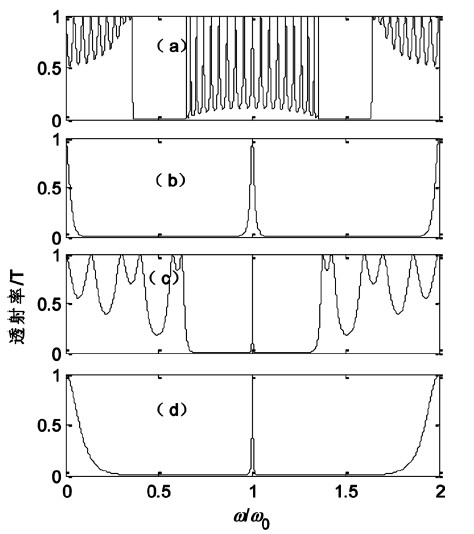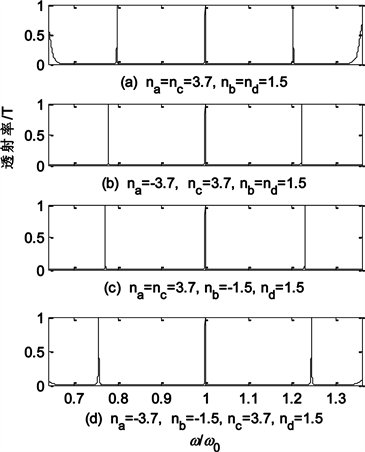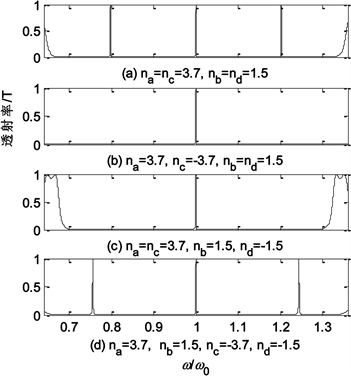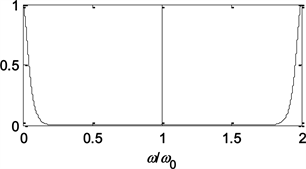# 含负折射率材料光量子阱透射谱对比研究Comparative Study on Transmission Spectrum of Optical Quantum Well Containing Negative Refractive Index Materials

DOI: 10.12677/OE.2019.92016, PDF, HTML, XML, 下载: 327  浏览: 742  科研立项经费支持

Abstract: The transmission matrix method is used to study the transmission characteristics of a one-dimen- sional symmetric structured optical quantum well (AB)m(CDDC)n(BA)m constructed with materials with positive and negative refractive index, and compare with the full positive refractive index materials. The results show that when the barrier layer (AB)m(BA)m of the optical quantum well is a mixed structure of positive and negative refractive index materials, the three transmission peaks of the transmission spectrum are narrower than the transmission peak of the optical quantum well of the full positive refractive index material, and the forbidden band becomes wider. When the well layer (CDDC)n is a material mixing structure, the transmission spectrum only shows a transmission peak at the center frequency position, and the transmission peak becomes wider; when the barrier layer and the well layer are simultaneously mixed structures, the transmission spectrum is only one transmission peak appears at the center frequency position, and the transmission peak becomes narrower, and the forbidden band becomes significantly wider. When the barrier layer or the well layer is completely negatively refractive, the transmission spectrum exhibits transmission peaks, the transmission peaks become wider, and the forbidden band widening. The study of the transmission spectrum characteristics of the optical quantum well constructed with a mixture of positive and negative refractive index materials provides a theoretical basis for the design and manufacture of new optical devices.

1. 引言

20世纪以来，光电材料一直作为科研领域的前沿科学，成为众多专家学者研究的热门。其中光子晶体由于其具有传统的光学材料所没有的光波控制特性，可以用来制作各种全新的高性能光学器件，拓宽了光电子技术的应用范围，在通讯、医疗、军事等领域被视为最具潜力的新型材料之一。随着光子晶体研究的不断深入，普通的光子晶体能带结构已经不能满足人们的理论及实际生产需要，即对光子晶体材料提出来更高的要求，负折射率材料构成的光子晶体开始进入研究者们的视野。所谓的负折射率材料(Negative index materials)是指的介电常数和渗透率都是负的介质材料，1968年Victor Veselago发表文章理论上假设负折射率材料的存在，直到2000年和2001年，第一次证明有负折射率的人工材料出现，光波在此类介质中相速度和群速度方向相反，即能量传播的方向和相位传播的方向相反，频移情况呈逆多普勒效应。利用负折射率材料的特殊性，研究者们通过构建光量子阱等特殊的光子晶体结构来改变光在光子晶体中的传输特性，达到操纵光波传输的目的，这些光量子阱在光的传输上显示出其特有的性质，但对负折射率材料构建的光量子阱的研究还较为稀缺，本文利用负折射率材料合理构建光量子阱，并对其传输特性进行研究，进一步丰富光子晶体的研究，同时利用这些特性设计和制造新型的滤波器、激光二极管、激光器和光放大器等提供理论基础。

2. 研究模型和方法

$\left[\begin{array}{c}{E}_{Ι}\\ {H}_{Ι}\end{array}\right]=M\left[\begin{array}{c}{E}_{Ι}{}_{Ι}\\ {H}_{Ι}{}_{Ι}\end{array}\right]=\left[\begin{array}{cc}\mathrm{cos}\delta & -\frac{i}{{\eta }_{b}}\mathrm{sin}\delta \\ -i\eta \mathrm{sin}\delta & \mathrm{cos}\delta \end{array}\right]\left[\begin{array}{c}{E}_{Ι}{}_{Ι}\\ {H}_{Ι}{}_{Ι}\end{array}\right]$

$\left[\begin{array}{c}{E}_{Ι}\\ {H}_{Ι}\end{array}\right]=\left({M}_{N}\right)\left[\begin{array}{c}{E}_{N+1}\\ {H}_{N+1}\end{array}\right]={M}_{1}{M}_{2}\cdot \cdot \cdot {M}_{N}\left[\begin{array}{c}{E}_{N+1}\\ {H}_{N+1}\end{array}\right]=M\left[\begin{array}{c}{E}_{N+1}\\ {H}_{N+1}\end{array}\right]=\left[\begin{array}{cc}A& B\\ C& D\end{array}\right]\left[\begin{array}{c}{E}_{N+1}\\ {H}_{N+1}\end{array}\right]$Figure 1. Transmission of (CDDC)10 and(AB)3(BA)3

3. 计算结果与分析

3.1. 垒层含负折射率材料时光量子阱的透射特性Figure 2. Transmission of (AB)3(CDDC)2(BA)3 when the barrier layer is a mixed structure

3.2. 阱层含负折射率材料时光量子阱的透射特性Figure 3. Transmission of (AB)3(CDDC)2(BA)3 when the well layer is a mixed structure

3.3. 阱层和垒层同时含负折射率材料时光量子阱的透射特性Figure 4. Transmission of (AB)3(CDDC)2(BA)3 when na = nc = −3.7, nb = nd = 1.5

4. 结论

1) 当的阱层全部为正折射率材料，垒层为混合结构时，量子阱关于频率中心对称出现3条缺陷模透射峰，较全正折射率材料量子阱，其透射峰更窄，禁带宽度更大。

2) 当垒层全部为正折射率材料，阱层为正混合结构时，量子阱在频率中心只出现一个透射峰，较正折射率材料量子阱，其透射峰更宽。

3) 当垒层或阱层全部为负折射率材料时，量子阱频率中心对称出现3条缺陷模透射峰，较正折射率材料量子阱，其透射峰更宽，禁带宽度更大。

4) 当的垒层或阱层均为正负折射率材料混合结构时，量子阱在频率中心只出现一个精细透射峰，较正折射率材料量子阱，其透射峰更窄，禁带宽度明显增大。

  Yablonovitch, E. (1987) Inhibited Spontaneous Emission in Solid-State Physics and Electronics. Physical Review Letters, 58, 2059-2061. https://doi.org/10.1103/PhysRevLett.58.2059  John, S. (1987) Strong Localization of Photons in Certain Disordered Dielectric Superlattices. Physical Review Letters, 58, 2486-2489. https://doi.org/10.1103/PhysRevLett.58.2486  苏安, 高英. 含双负介质一维光子晶体量子阱的透射谱研究[J]. 量子电子学报, 2010, 27(5): 1007-5461.  苏安, 高英俊, 蒙成举. 双重势垒一维光子晶体量子阱内部局域电场分布[J]. 光子学报, 2014, 43(2): 0216002.  苏安, 蒙成举, 高英俊. 激活性杂质对光子晶体量子阱滤波器特性的调制[J]. 中国激光, 2014, 41(3): 0306001.  苏安, 蒙成举, 高英俊. 实现高品质滤波功能的一维光子晶体量子阱滤波器[J]. 中国激光, 2013, 40(10): 1006001.  苏安, 彭金松, 潘继环, 等. 结构周期数对光子晶体量子阱透射品质影响的研究[J]. 广西物理, 2013, 34(13): 1003-7551.  苏安, 蒙成举, 高英俊. 左手介质对一维光量子阱透射谱的影响[J]. 激光与光电子学进展, 2013, 50(1): 012302.  许江勇, 苏安, 潘继环, 等. 双负介质对一维光子晶体量子阱透射谱的影响[J]. 激光与红外, 2013, 42(8): 1007-2276.  潘继环, 苏安, 蒙成举. 双负介质对光子晶体透射能带谱的简并效应研究[J]. 光学技术, 2014, 40(3): 1002-1582.  潘继环. 双正、双负和单负介质光子晶体能带的比较研究[J]. 河池学院学报, 2014, 34(2): 1672-9021.  苏安, 李忠海, 莫传文, 等. 光子晶体滤波器的滤波品质调制因素研究[J]. 河池学院学报, 2014, 34(5): 78-82.  潘继环, 苏安, 唐秀福, 等. 缺陷奇偶性对光子晶体光传输特性的影响[J]. 激光与红外, 2015, 45(6): 705-709.  韦吉爵, 李忠海, 莫传文, 等. 两种因素对光子晶体缺陷模的影响[J]. 河池学院学报, 2015, 35(2): 40-44.  潘继环, 李志海, 黄金玉. 介质光学厚度对双负材料光子晶体透射峰带宽的调制[J]. 河池学院学报, 2015, 35(2): 1672-9021.  唐秀福. 光子晶体研究方法对比与实例分析[J]. 河池学院学报, 2015, 35(2): 45-49.